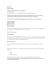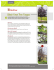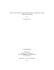# Sheet 3

## Transcription

Sheet 3
```Introduction to Knot Theory FS 2015 – Meike Akveld
Exercisesheet 3: Simple knot invariants
1. Prove that the unknotting number u(K) is finite.
2. (a) Prove that for any oriented link L the linking number lk(L) is an
integer.
(b) Choose an orientation for the following link, the Borromean rings,
change if you reverse one, two or all three of the components?
3. Prove that c(K) and u(K) are subadditive invariants i.e.
c(K1 #K2 ) ≤ c(K1 ) + c(K2 )
and likewise for u(K).
Remark: It is conjectured that both these invariants are in fact additive,
i.e. that equality holds above, but no one was able to prove that so far.
4. Prove that the linking number lk(L) is invariant under Reidemeister move
3.
5. Draw a picture to show that there exist oriented 2-component links with
linking number n, for any integer n.
6. Prove that if the orientation on one component of a 2-component oriented
link L is reversed then its linking number is negated. Moreover, determine
the linking number of the mirror image of L.
7. Prove that any knot diagram has at least three 3-colourings and hence
τ3 (D) ≥ 3.
8. Show that τ3 (L) is invariant under Reidemeister move 3.
9. Compute τ3 of the unknot and τ3 (51 ) – what do you observe? Can we use
τ3 to distinguish the two knots with crossing number 5?
the unlink, but that τ3 succeeds.
1
Introduction to Knot Theory FS 2015 – Meike Akveld
11. (a) Show that τ3 (K) is always divisible by 3.
(b) Show that τ3 (K) is always a power of 3.
(c) Show that τ3 (K) − 3 is always divisible by 3!.
12. Show that τ3 cannot distinguish a knot from its mirror image.
13. Use τ3 (K1 #K2 ) = 31 τ3 (K1 )τ3 (K2 ) to prove that there are infinitely many
distinct knots.
14. Calculate τ3 (L) for the following two two-component links. Can you decide
whether these links are equivalent or not?
15. Determine for each of the following statements whether it is true or false.
(a) If K1 and K2 are knots with τ3 (K1 ) = τ3 (K2 ) then K1 is equivalent
to K2 .
(b) If K1 and K2 are equivalent knots, then τ3 (K1 ) = τ3 (K2 ).
(c) If an oriented link has lk(L) = 0, then L is equivalent to the unlink.
(d) There is no knot with τ3 (K) = 6.
(e) For any knot τ3 (K) = −τ3 (K).
(f) The function f defined on knot diagrams by setting
f (D) = number of crossings on D
defines an invariant of knots.
(g) For any n, the number of knots with crossing number at most n is
finite.
(h) For any n, the number of knots with unknotting number at most n
is finite.
(i) For any oriented knot K, τ5 (rK) = τ5 (K).
2
Introduction to Knot Theory FS 2015 – Meike Akveld
(j) For any knot τ2 (K) = 2.
16. Show that the equation that needs to be satisfied for 3-colourability is
equivalent to the general equation for p-colourability.
17. Show that τ2 equals 2 to the power of the number of components of the
link and is therefore not very interesting.
18. Let τ5 be the number of 5-colourings of a link.
(a) Use τ5 to show that the figure-eight knot 41 is non-trivial.
(b) Calculate τ5 (41 #41 )
(c) Suppose the diagram below allows non-trivial 5-colourings with the
colours a, b, c and d emerging at the ends as shown. Show that a = c
and b = d.
a
c
b
d
19. Calculate the number of 5- and 7-colourings of the knot 71 .
20. Suppose K+ and K− are two knots having diagrams D+ and D− , which
are identical except at one crossing, as shown below.
D−
D+
(a) Prove that τp (K+ ) and τp (K− ) are either equal or one is p times the
other.
(b) Deduce that the unknotting number of a knot that satisfies
u(K) ≥ logp (τp (K)) − 1
Due Date: 24.03.2015
3
```

### Boy Scout Rope Work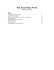### H T S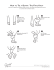### Make a Sisters 8 Friendship Bracelet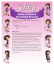### Prayer to Mary, Undoer of Knots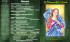### Colligo Marine recommended Stopper Knot Stevedores Knot or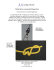### Macramé plant hanger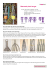### Essentlal Ties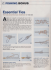### Class A Knot Tying - Fairbanks Girl Scouts